Optimization with constraints (constraints) is a process of optimizing an objective function with respect to some variables under certain conditions. The objective function is either a cost function that should be minimized, or a reward function that should be maximized.

Basically, a constraint is a hard limit placed on the value of a variable, which prevents us from going indefinitely in certain directions.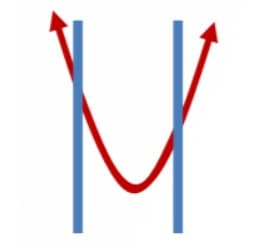In hard constraints, the conditions that variables must satisfy are specified, in soft constraints, the variables’ values are penalized in the objective function if and to the extent that the conditions on the variables are not satisfied.

The constrained-optimization problem is a generalization of the classic constraint-satisfaction problem model.

There is also unconstrained optimization, which either has no boundaries or has soft boundaries, but that is a topic for later.

In mathematical optimization, there are two ways to find the optimum (numerically):

1. Direct Search: Here, we use only the function values at a given point to find the optimum. It works by comparing the function values in a neighborhood of a point and moving in the direction that results in a decrease in the function value (for minimization problems). Direct Search methods are commonly used when the function is discontinuous, and hence the derivative is not available.
2. Gradient-based methods: We use the first and second-order derivatives to locate the optima. Methods that consider gradient information have the advantage of convergence faster.

There are two types of Constrained optimization

#### Equality constraints

A general constrained minimization problem is written as follows: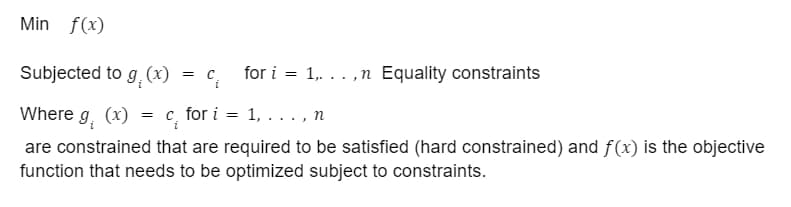#### Inequality Constraints

The Lagrange multiplier technique can be used when some of the constraints are inequalities, but it must be carefully checked in its details.

A general constrained minimization problem is written as follows: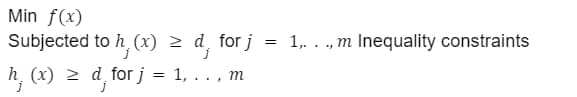Constraints that must be satisfied (hard constraints) and is the objective function that must be optimized by taking into account constraints.

The complexity of a problem can be described in terms of geometric optimality conditions, Fritz John conditions and Karush-Kuhn-Tucker conditions, under which simple problems may be solvable.

#### Linear

With linear functions, the optimum values can only occur at the boundaries.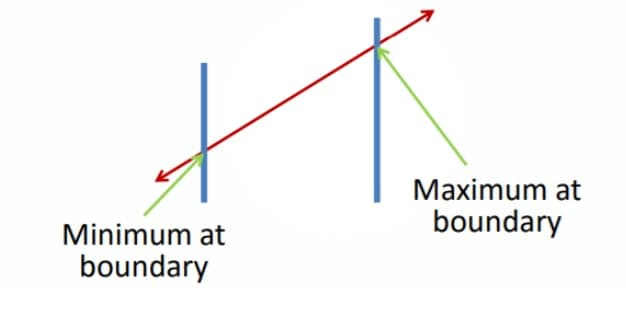#### Non-Linear

With nonlinear functions, the optimum values can either occur at the boundaries or between them.#### Active Constrained

An optimal solution that lies at the intersection point of two constraints causes both of those constraints to be considered active.#### Inactive Constrained

If any of the constraint lines do not pass through the optimal point, those constraints are called inactive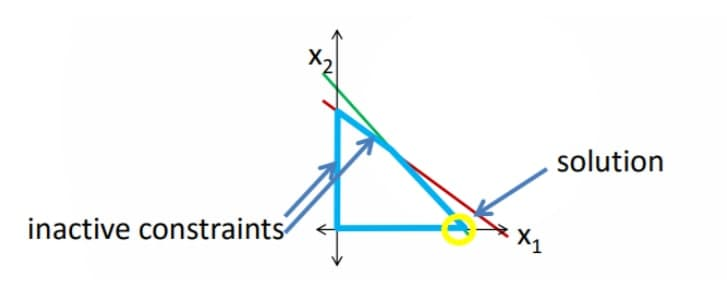Here we conclude this topic and hope you learned something new today and hope to see you soon. Thank You